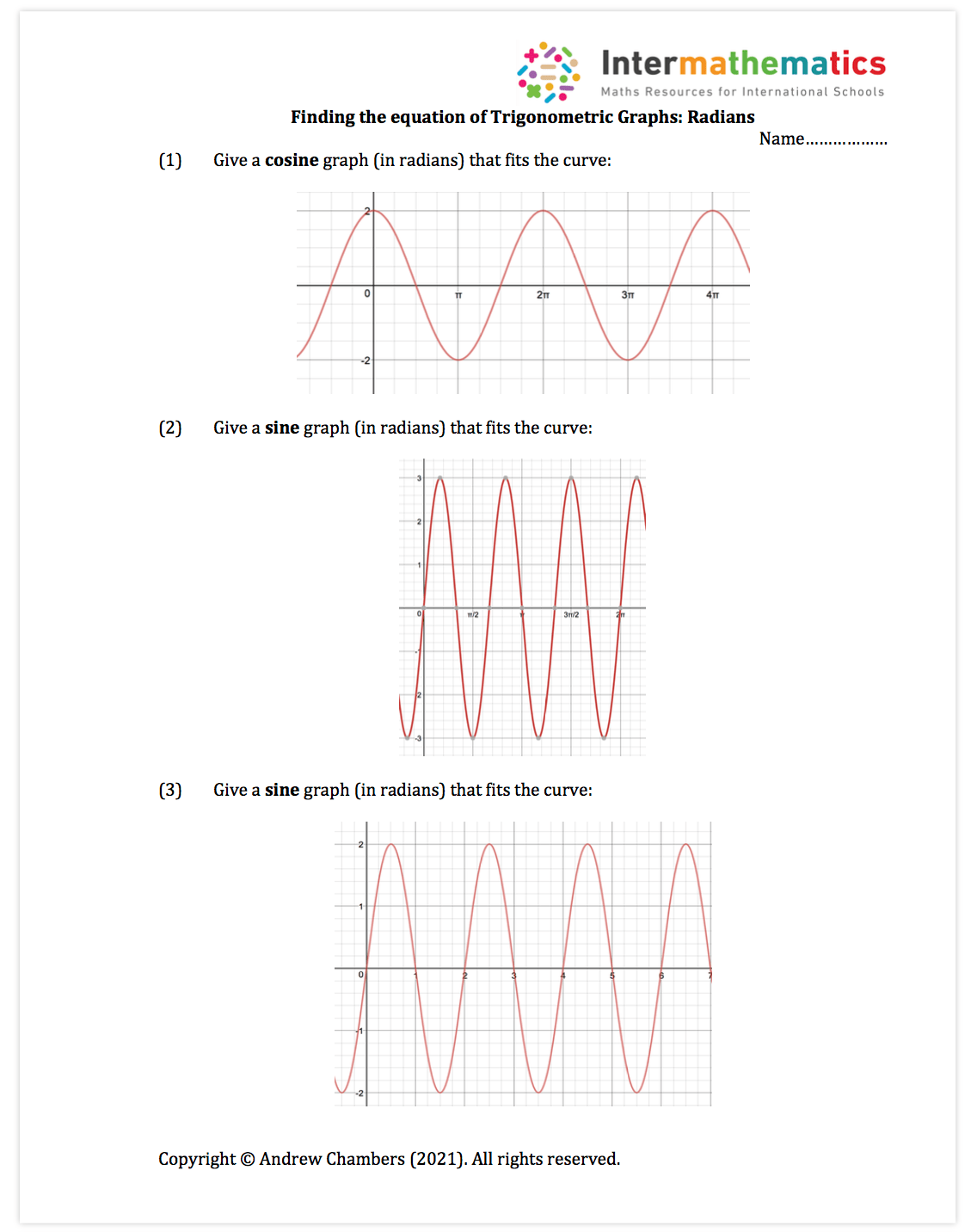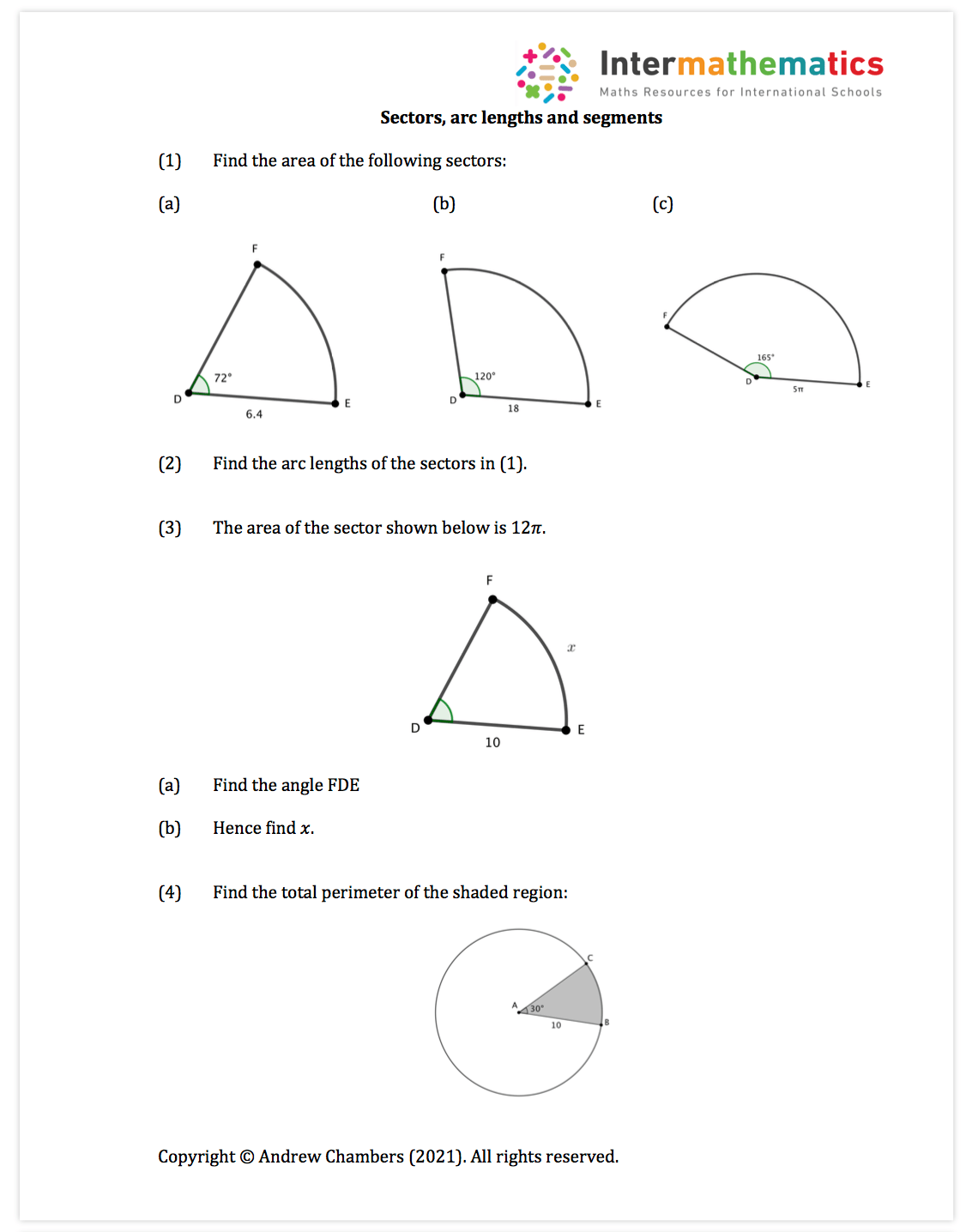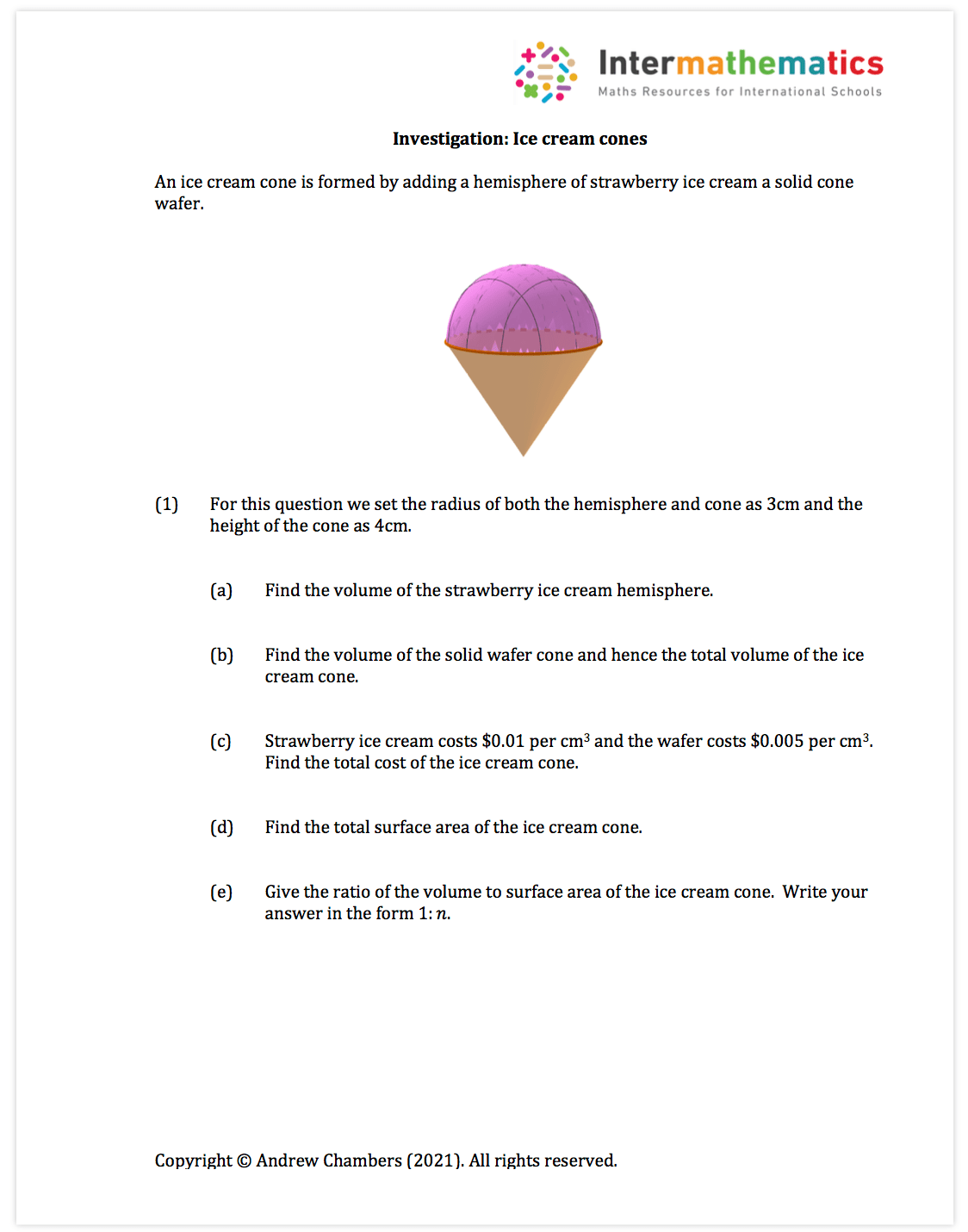# Trigonometry Free Content

Trigonometry Content: Content to help with trig triangles, exact values, trig graphs, transformations, solving trig equations, use of trig identities.

Trigonometry content in premium set: 25 worksheets (around 55 pdf pages of questions and around 80 pdf pages of fully typed solutions)

This unit mixes up algebraic skills, calculator skills and non calculator skills.  I’ve used Desmos and Geogebra extensively in this section.

Example trig graphs worksheet:Example trigonometric graph investigation:Example solving trig equations activity (treasure hunt):Example sectors and segments worksheet:Paper 3 investigation example:3D volumes worksheet example: